•#### Learning Bayesian Models with R#### Overview of this book

Learning Bayesian Models with RCreditswww.PacktPub.comPrefaceFree Chapter
Introducing the Probability TheoryThe R EnvironmentIntroducing Bayesian InferenceMachine Learning Using Bayesian InferenceBayesian Regression ModelsBayesian Classification ModelsBayesian Models for Unsupervised LearningBayesian Neural NetworksBayesian Modeling at Big Data ScaleIndex## Bayesian mixture models

In general, a mixture model corresponds to representing data using a mixture of probability distributions. The most common mixture model is of the following type: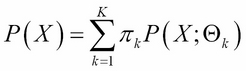Here,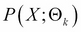is a probability distribution of X with parameters, and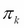represents the weight for the kth component in the mixture, such that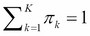. If the underlying probability distribution is a normal (Gaussian) distribution, then the mixture model is called a Gaussian mixture model (GMM). The mathematical representation of GMM, therefore, is given by: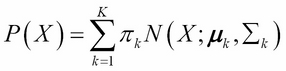Here, we have used the same notation, as in previous chapters, where X stands for an N-dimensional data vector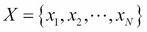representing each observation and there are M such observations in the dataset.

A mixture model such as this is suitable for clustering when the clusters have overlaps. One of the applications of GMM is in computer vision. If one wants to track moving objects in a video, it is useful to subtract the background image. This is called background subtraction or...